Successfully reported this slideshow.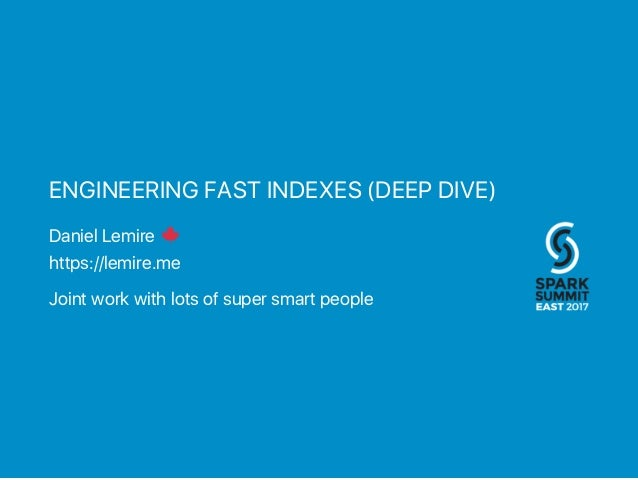Upcoming SlideShare
×

of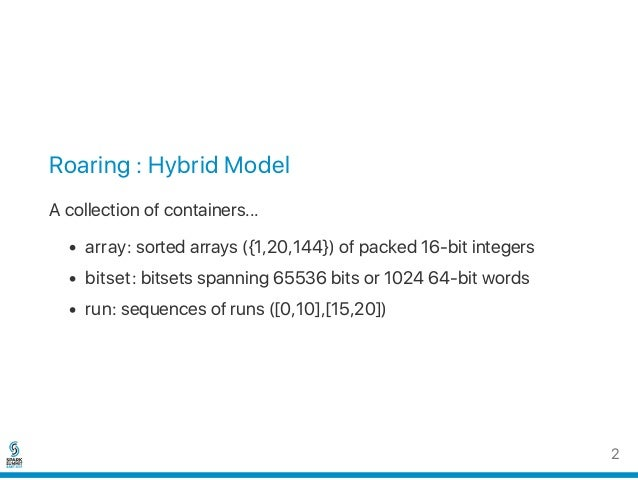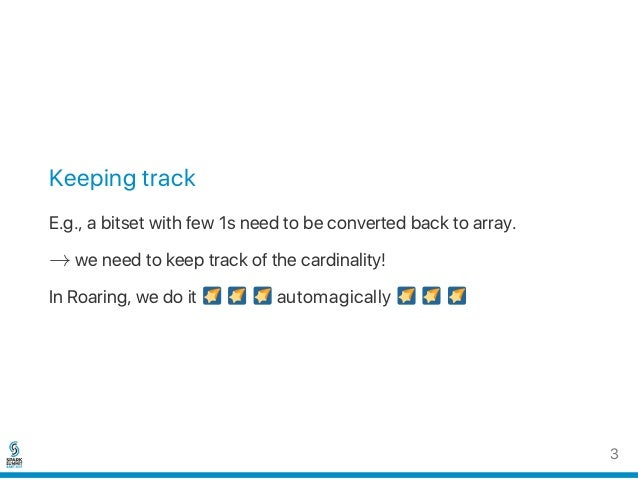Upcoming SlideShare
Engineering fast indexes
Next

Share

# Engineering fast indexes (Deepdive)

Contemporary computing hardware offers massive new performance opportunities. Yet high-performance programming remains a daunting challenge.

See all

See all

### Engineering fast indexes (Deepdive)

1. 1. ENGINEERING FAST INDEXES (DEEP DIVE) Daniel Lemire https://lemire.me Joint work with lots of super smart people
2. 2. Roaring : Hybrid Model A collection of containers... array: sorted arrays ({1,20,144}) of packed 16‑bit integers bitset: bitsets spanning 65536 bits or 1024 64‑bit words run: sequences of runs ([0,10],[15,20]) 2
3. 3. Keeping track E.g., a bitset with few 1s need to be converted back to array. → we need to keep track of the cardinality! In Roaring, we do it automagically 3
4. 4. Setting/Flipping/Clearing bits while keeping track Important : avoid mispredicted branches Pure C/Java: q = p / 64 ow = w[ q ]; nw = ow | (1 << (p % 64) ); cardinality += (ow ^ nw) >> (p % 64) ; // EXTRA w[ q ] = nw; 4
5. 5. In x64 assembly with BMI instructions: shrx %, %[p], %[q] // q = p / 64 mov (%[w],%[q],8), %[ow] // ow = w [q] bts %[p], %[ow] // ow |= ( 1<< (p % 64)) + flag sbb \$-1, %[cardinality] // update card based on flag mov %[load], (%[w],%[q],8) // w[q] = ow  sbb is the extra work 5
6. 6. For each operation union intersection difference ... Must specialize by container type: array bitset run array ? ? ? bitset ? ? ? run ? ? ? 6
7. 7. High‑level API or Sipping Straw? 7
8. 8. Bitset vs. Bitset... Intersection: First compute the cardinality of the result. If low, use an array for the result (slow), otherwise generate a bitset (fast). Union: Always generate a bitset (fast). (Unless cardinality is high then maybe create a run!) We generally keep track of the cardinality of the result. 8
9. 9. Cardinality of the result How fast does this code run? int c = 0; for (int k = 0; k < 1024; ++k) { c += Long.bitCount(A[k] & B[k]); } We have 1024 calls to  Long.bitCount . This counts the number of 1s in a 64‑bit word. 9
10. 10. Population count in Java // Hacker`s Delight int bitCount(long i) { // HD, Figure 5-14 i = i - ((i >>> 1) & 0x5555555555555555L); i = (i & 0x3333333333333333L) + ((i >>> 2) & 0x3333333333333333L); i = (i + (i >>> 4)) & 0x0f0f0f0f0f0f0f0fL; i = i + (i >>> 8); i = i + (i >>> 16); i = i + (i >>> 32); return (int)i & 0x7f; } Sounds expensive? 10
11. 11. Population count in C How do you think that the C compiler  clang compiles this code? #include <stdint.h> int count(uint64_t x) { int v = 0; while(x != 0) { x &= x - 1; v++; } return v; } 11
12. 12. Compile with  -O1 -march=native on a recent x64 machine: popcnt rax, rdi 12
13. 13. Why care for  popcnt ?  popcnt : throughput of 1 instruction per cycle (recent Intel CPUs) Really fast. 13
14. 14. Population count in Java? // Hacker`s Delight int bitCount(long i) { // HD, Figure 5-14 i = i - ((i >>> 1) & 0x5555555555555555L); i = (i & 0x3333333333333333L) + ((i >>> 2) & 0x3333333333333333L); i = (i + (i >>> 4)) & 0x0f0f0f0f0f0f0f0fL; i = i + (i >>> 8); i = i + (i >>> 16); i = i + (i >>> 32); return (int)i & 0x7f; } 14
15. 15. Population count in Java! Also compiles to  popcnt if hardware supports it \$ java -XX:+PrintFlagsFinal | grep UsePopCountInstruction bool UsePopCountInstruction = true But only if you call it from  Long.bitCount  15
16. 16. Java intrinsics  Long.bitCount ,  Integer.bitCount   Integer.reverseBytes ,  Long.reverseBytes   Integer.numberOfLeadingZeros ,  Long.numberOfLeadingZeros   Integer.numberOfTrailingZeros ,  Long.numberOfTrailingZeros   System.arraycopy  ... 16
17. 17. Cardinality of the intersection How fast does this code run? int c = 0; for (int k = 0; k < 1024; ++k) { c += Long.bitCount(A[k] & B[k]); } A bit over ≈ 2 cycles per pair of 64‑bit words. load A, load B bitwise AND  popcnt  17
18. 18. Take away Bitset vs. Bitset operations are fast even if you need to track the cardinality. even in Java e.g.,  popcnt overhead might be negligible compared to other costs like cache misses. 18
19. 19. Array vs. Array intersection Always output an array. Use galloping O(m log n) if the sizes differs a lot. int intersect(A, B) { if (A.length * 25 < B.length) { return galloping(A,B); } else if (B.length * 25 < A.length) { return galloping(B,A); } else { return boring_intersection(A,B); } } 19
20. 20. Galloping intersection You have two arrays a small and a large one... while (true) { if (largeSet[k1] < smallSet[k2]) { find k1 by binary search such that largeSet[k1] >= smallSet[k2] } if (smallSet[k2] < largeSet[k1]) { ++k2; } else { // got a match! (smallSet[k2] == largeSet[k1]) } } If the small set is tiny, runs in O(log(size of big set)) 20
21. 21. Array vs. Array union Union: If sum of cardinalities is large, go for a bitset. Revert to an array if we got it wrong. union (A,B) { total = A.length + B.length; if (total > DEFAULT_MAX_SIZE) {// bitmap? create empty bitmap C and add both A and B to it if (C.cardinality <= DEFAULT_MAX_SIZE) { convert C to array } else if (C is full) { convert C to run } else { C is fine as a bitmap } } otherwise merge two arrays and output array } 21
22. 22. Array vs. Bitmap (Intersection)... Intersection: Always an array. Branchy (3 to 16 cycles per array value): answer = new array for value in array { if value in bitset { append value to answer } } 22
23. 23. Branchless (3 cycles per array value): answer = new array pos = 0 for value in array { answer[pos] = value pos += bit_value(bitset, value) } 23
24. 24. Array vs. Bitmap (Union)... Always a bitset. Very fast. Few cycles per value in array. answer = clone the bitset for value in array { // branchless set bit in answer at index value } Without tracking the cardinality ≈ 1.65 cycles per value Tracking the cardinality ≈ 2.2 cycles per value 24
25. 25. Parallelization is not just multicore + distributed In practice, all commodity processors support Single instruction, multiple data (SIMD) instructions. Raspberry Pi Your phone Your PC Working with words x × larger has the potential of multiplying the performance by x. No lock needed. Purely deterministic/testable. 25
26. 26. SIMD is not too hard conceptually Instead of working with x + y you do (x , x , x , x ) + (y , y , y , y ). Alas: it is messy in actual code. 1 2 3 4 1 2 3 4 26
27. 27. With SIMD small words help! With scalar code, working on 16‑bit integers is not 2 × faster than 32‑bit integers. But with SIMD instructions, going from 64‑bit integers to 16‑bit integers can mean 4 × gain. Roaring uses arrays of 16‑bit integers. 27
28. 28. Bitsets are vectorizable Logical ORs, ANDs, ANDNOTs, XORs can be computed fast with Single instruction, multiple data (SIMD) instructions. Intel Cannonlake (late 2017), AVX‑512 Operate on 64 bytes with ONE instruction → Several 512‑bit ops/cycle Java 9's Hotspot can use AVX 512 ARM v8‑A to get Scalable Vector Extension... up to 2048 bits!!! 28
29. 29. Java supports advanced SIMD instructions \$ java -XX:+PrintFlagsFinal -version |grep "AVX" intx UseAVX = 2 29
30. 30. Vectorization matters! for(size_t i = 0; i < len; i++) { a[i] |= b[i]; } using scalar : 1.5 cycles per byte with AVX2 : 0.43 cycles per byte (3.5 × better) With AVX‑512, the performance gap exceeds 5 × Can also vectorize OR, AND, ANDNOT, XOR + population count (AVX2‑Harley‑Seal) 30
31. 31. Vectorization beats  popcnt  int count = 0; for(size_t i = 0; i < len; i++) { count += popcount(a[i]); } using fast scalar (popcnt): 1 cycle per input byte using AVX2 Harley‑Seal: 0.5 cycles per input byte even greater gain with AVX‑512 31
32. 32. Sorted arrays sorted arrays are vectorizable: array union array difference array symmetric difference array intersection sorted arrays can be compressed with SIMD 32
33. 33. Bitsets are vectorizable... sadly... Java's hotspot is limited in what it can autovectorize: 1. Copying arrays 2. String.indexOf 3. ... And it seems that  Unsafe effectively disables autovectorization! 33
34. 34. There is hope yet for Java One big reason, today, for binding closely to hardware is to process wider data flows in SIMD modes. (And IMO this is a long‑term trend towards right‑sizing data channel widths, as hardware grows wider in various ways.) AVX bindings are where we are experimenting, today (John Rose, Oracle) 34
35. 35. Fun things you can do with SIMD: Masked VByte Consider the ubiquitous VByte format: Use 1 byte to store all integers in [0, 2 ) Use 2 bytes to store all integers in [2 , 2 ) ... Decoding can become a bottleneck. Google developed Varint‑GB. What if you are stuck with the conventional format? (E.g., Lucene, LEB128, Protocol Buffers...) 7 7 14 35
36. 36. Masked VByte Joint work with J. Plaisance (Indeed.com) and N. Kurz. http://maskedvbyte.org/ 36
37. 37. Go try it out! Fully vectorized Roaring implementation (C/C++): https://github.com/RoaringBitmap/CRoaring Wrappers in Python, Go, Rust... 37
•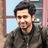#### vinothchandar

Feb. 11, 2017
•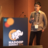#### maropu0804

Feb. 10, 2017
•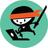#### NELLAIVIJAY1

Feb. 10, 2017
•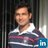#### KishoreGopalakrishna

Feb. 10, 2017

Total views

7,447

On Slideshare

0

From embeds

0

Number of embeds

66

25

Shares

0